# Cholesky Factorization

In the cases when the system matrix A is symmetric positive definite (SPD) the Cholesky factorization can be used to decompose the system matrix A into a product of two triangular matrices

A = L*L’
where L is a lower triangular factor and L’ is its transpose. Whenever applicable, the Cholesky algorithm is advantageous to other direct methods of solving linear systems of equations.

1. Only the lower triangular part of matrix A needs to be computed and assembled in the memory.

2. The Cholesky algorithm only computes the lower triangular factor, which roughly halves the number of operations and the amount of memory required.

3. Cholesky factorization is fully deterministic and does not depend on the matrix entries, i.e., it does not require pivoting. Consequently, symbolic factorization can be used to determine the exact non-zero structure of the factor based on the non-zero structure of A before the actual factorization is computed. This improves the performance significantly because required memory structures can be pre-allocated beforehand.

MATLAB is distributed with CHOLMOD, a fast Cholesky algorithm implementation by Tim Davis . Factorization of a symmetric sparse matrix A, of which only the lower triangular part is stored in the memory, is invoked with the following syntax

L = chol(A,’lower’);
Remark: For MATLAB versions older than 2011a it is recommended to download the newest version of CHOLMOD directly and compile it to have a better performing Cholesky solver. MILAMIN2 will attempt to do this automatically.

### Sequential Factorization Performance

The time required to compute the factor is determined by two major factors: the system size n, which determines the factorization operation count, and the computational capabilities of the computer. As shown in the Reordering section, using METIS reordering the number FLOPs required to compute the factor is proportional to n1.56 and n2.06 for 2D and 3D problems, respectively.

#### 2D Problems

Assuming the computer used to perform the calculations has a performance of X floating point operations per second (FLOP/s), the time required to factorize a matrix for a 2D thermal problem can be estimated as

t2D(n) = C * n1.56 / X
where C is depends on the element type used and the number of degrees of freedom per node. In practice the above performance model is modified by the fact that the computational performance during factorization also depends on the system size n, i.e., X=X(n), see Figure 1.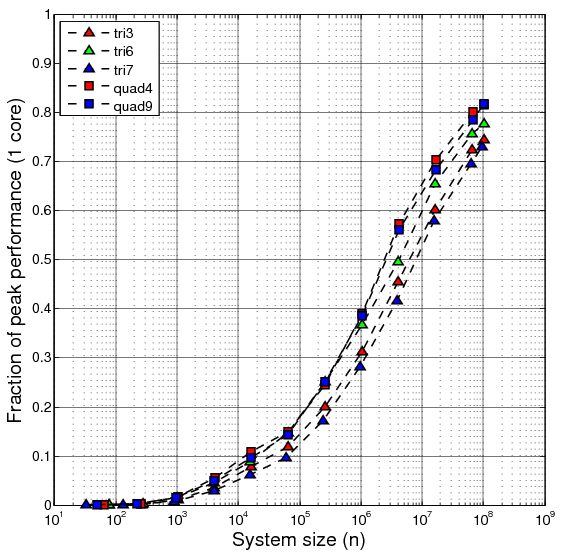Figure 1. Efficiency on 1 CPU core (or fraction of peak FLOP/s performance) of Cholesky factorization for matrices resulting from 2D thermal problems discretized using a variety of elements. The computer used in the tests was Valinor - a Dell server with Xeon E7- 4870 CPUs (see Tested Computer Architectures section). Peak per-core performance of 11.2 GFLOP/s.

One conclusion that can be drawn from this figure is that the CPU can compute the factorization more efficiently for larger matrices. There are a number of reasons for this behavior.

1. The performance of Cholesky factorization largely depends on the performance of BLAS Level 3 dense matrix-matrix multiplication (GEMM) and triangular solve with multiple right-hand sides (TRSM) implementations. On modern multi-core CPU architectures BLAS L3 is often advertised to run close to (more than 90%) the peak multi-core performance for large enough matrices. However, for small matrices the performance is well below the peak of even a single CPU core. Thus, performance of the Cholesky solver depends on the types of dense matrices being multiplied. (Note that in tests presented in Figure 1. we used a single-threaded BLAS. Parallel performance is considered later.)

2. During sparse Cholesky factorization BLAS L3 routines are executed for a variety of dense matrices with different dimensions. In the Reordering section it has been demonstrated that the number of non-zeros in the factor grows like system size n to some power greater than 1. In fact, it can be observed that for the growing system size n the dimensions of the matrices that need to be multiplied also grow. For large enough n BLAS L3 calls for the largest matrices starts to dominate the total execution time, hence the improved overall efficiency of Cholesky factorization.

3. For larger systems the overhead connected with initialization of the Cholesky algorithm is relatively smaller. In the symbolic factorization stage the number of non-zero entries in every row of the factor is computed. The complexity of symbolic factorization is linear with respect to the system size. Hence, the overhead introduced by this stage disappears with the system size.

Figure 1 demonstrates that for 2D thermal problems the factorization achieves only around 50% of the peak CPU performance for systems as large as 4 million degrees of freedom. 70-80% of the peak is achieved for systems with roughly 100 million degrees of freedom. Note that the performance is better for quad elements and the 6-node triangular element, while lower performance is observed for the 3- and 7-node elements.

#### 3D Problems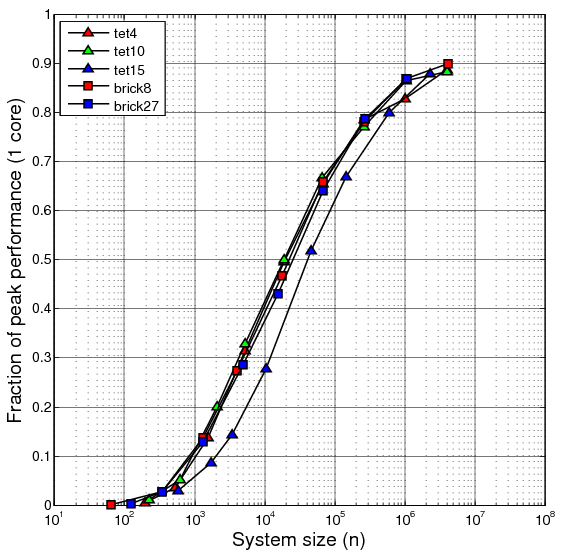Figure 1. Efficiency on 1 CPU core (or fraction of peak FLOP/s performance) of Cholesky factorization for matrices resulting from 3D thermal problems discretized using a variety of elements. The computer used in the tests was Valinor - a Dell server with Xeon E7- 4870 CPUs (see Tested Computer Architectures section). Peak per-core performance of 11.2 GFLOP/s.

Figure 2 shows factorization efficiency results for 3D thermal problems and various element types. Clearly, factorization efficiency for 3D problems is significantly better than for 2D. On the tested computer 50% of peak FLOP/s performance is achieved for problems with approximately 104 mesh nodes. For models with approximately 3-4 million nodes the solver runs at over 10 GFLOP/s, around 90% of a single core peak performance.

### Parallel Factorization Performance

The literature on parallel direct solvers for various types of linear systems of equations is vast. Obtaining a scalable and highly parallel direct solver implementation is quite challenging considering both the technical requirements an the complexity of the algorithms. The approach used in MATLAB can be implemented relatively easily on multi-core systems with shared memory. It is based on the observation that the Cholesky solver makes extensive use of the BLAS library, especially of the GEMM/TRSM implementations. Hence, even though the CHOLMOD solver used by MATLAB is sequential, it can be parallelized to some extent by providing a parallel BLAS implementation.

#### 2D Problems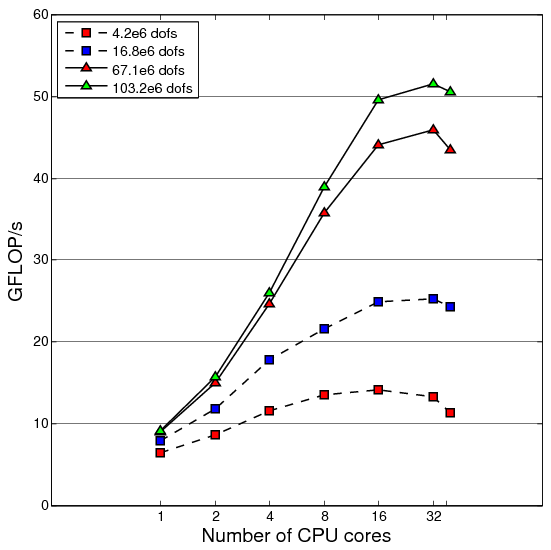Figure 3. Parallel factorization performance, 2D thermal problem, 4-node quad element.

Figure 3 presents the parallel performance of the Cholesky solver for a chosen 2D element on the 40-core Valinor SMP server. The peak performance of a single core on this computer is 11.2 GFLOP/s. For the largest studied system size (100 million dofs) the observed single core performance is roughly 9 GFLOP/s, 80% of peak (compare this to single core efficiency shown in Figure 1). Increasing the number of cores up to 16 results in some speedup. Further increase in the number of cores is ineffective or even degrades the performance. Similar behavior is observed for the smaller system sizes.

For the largest problem size the highest achieved performance of ~50 GFLOP/s is more than 5 times the single core performance. Considering that 16 cores were used to achieve the 5 times speedup it is clear that the per-core efficiency significantly degrades in the parallel run. Indeed, 16 cores have a peak performance of 16*11.2=179.2 GFLOP/s. Hence, the observed 50 GFLOP/s corresponds to around 28% of the theoretical peak, while the single-core efficiency was 80%.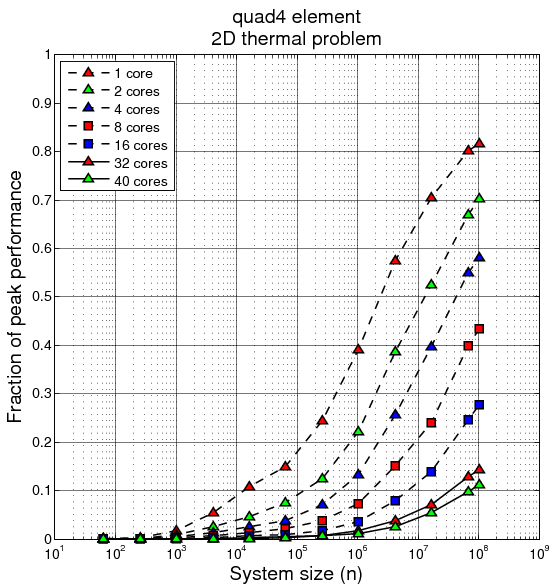Figure 4. Efficiency (fraction of peak multi-core performance) of parallel Cholesky factorization. Matrices resulting from 2D meshes using quad4 elements. Valinor server.

Figure 4 shows a systematic study of the efficiency of the parallel factorization for many 2D problem sizes and the 4-node quad element. Factorization efficiency defined as the fraction of the peak FLOP/s performance is shown for different number of CPU cores. For every parallel configuration the peak performance is calculated taking into account the number of cores actually used.

As also seen in Figure 1, the single core factorization efficiency increases with the system size reaching 80% for the largest studied systems. Figure 4 demonstrates that when factorizing a given system of equations using an increasing number of cores the efficiency quickly degrades. However, as in the sequential case, for a fixed number of cores the efficiency clearly increases with an increasing system size. One can now ask how fast does the system size n need to grow to assure the same factorization efficiency on increasing number of cores. For example, in the considered 2D case 50% efficiency is obtained on 1 core for a problem size of roughly 2*106 nodes. What is the problem size for which the Cholesky solver will run with 50% efficiency on 2 cores? Such relation between the problem size n and the number of cores is called isoefficiency function.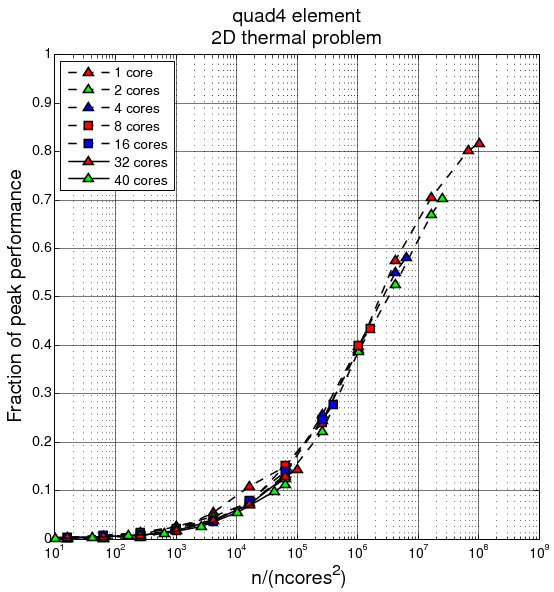Figure 5. Efficiency of parallel Cholesky factorization as a function of the system size scaled by the number of CPU cores squared. Matrices resulting from 2D meshes using quad4 elements. Valinor server.

Figure 5 presents the same performance results as Figure 4, but the X axis – the problem size n – is in this case scaled by the number of cores squared. All the lines for parallel configurations with different number of CPU cores plot on the same curve. This means that with the employed parallelization technique, for the studied architecture, and for the 2D thermal problems discretized using 4-node quad element

E = f(n/(ncores2))
where E denotes efficiency. The above relation also holds for other tested 2D elements. Since for the single core case ncores2=1 and hence E=f(n), Figure 1 in fact shows the isoefficiency functions for different 2D element types. The usefulness of the above considerations can be demonstrated by the following practical examples.

Example 1. Assuming we want to use the 32 cores on Valinor with 50% efficiency to solve a 2D thermal problem, what is the system size n required? From Figure 5 we see that for 50% efficiency

n/ncores2 = 2*106
Hence, if we use ncores=32

n = 2*106*322 = 2*109

Example 2. How much time will it take to factorize a system of size n=108 dofs using 32 CPU cores assuming METIS reordering is used?

n/ncores2 = 108/322 = 105
From Figure 5 we read that the expected factorization efficiency is in this case 18%. Hence, the expected factorization performance is roughly 0.18*32*11.2 = 64 GFLOP/s. From Figure 3 in the Reordering section we can infer that factorization of the given system will require around 5*1013 floating point operations. Hence, with the expected performance it will take roughly

t = 5*1013 [FLOP] / 64*109 [FLOP/s] = 781 [s]
The actual measured performance for the 4-node quad element and a system with 103 million dofs is 52 GFLOP/s and the factorization time on 32 CPU cores was 847 seconds.

#### 3D Problems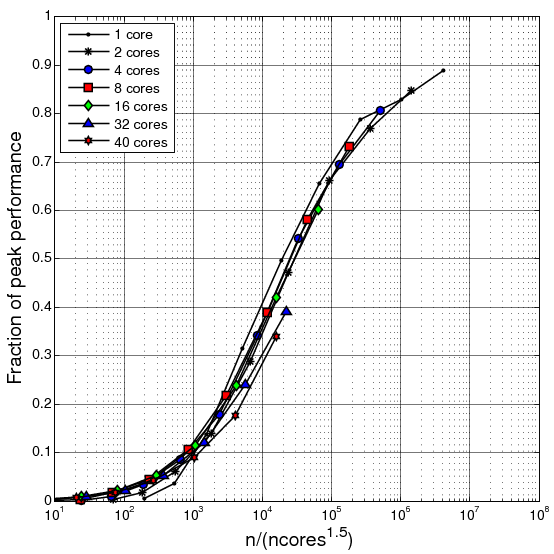Figure 6. Efficiency of parallel Cholesky factorization as a function of the system size scaled by the number of CPU cores in 1.5 power. Matrices resulting from 3D meshes using tet4 elements. Valinor server.

Figure 6 shows the isoefficiency function for the 4-node tetrahedral element. Parallel Cholesky solver is more scalable in 3D since in order to keep constant efficiency the problem needs to grow slower with the system size than in the 2D case

E = f(n/(ncores1.5))
or the system size needs to grow with the number of processors in power 1.5. These results are in agreement with other studies found in the literature on massively parallel sparse factorization .

Clearly, sparse factorization for 3D problems is both more efficient for smaller system sizes, and more amenable to parallelization than in the 2D case. However, as shown in the Reordering section, the operation count and the number of non-zero entries in the factor grows much faster for 3D problems.

Example 3. In Example 2. above we considered a 2D problem with 108 nodes. In this case factorization required approximately 1014 operations. For 3D problems similar number of operations is needed already for problems of size n=3*106. Using 32 CPU cores to factorize this problem yields

n/ncores1.5 = 3*106/(321.5) = 2*105
From Figure 6 we can see that in this case the expected factorization efficiency is around 75%, i.e., 265 GFLOP/s. This is more than 4 times faster than in the corresponding 2D case. However, in terms of the number of mesh nodes the 3D problem is 35 times smaller than its 2D counterpart. Consequently, in this example the 3D models are roughly 9 times more expensive per grid node.

### References

 http://www.cise.ufl.edu/research/sparse/SuiteSparse/
 A. Gupta, S. Koric, T. George, Sparse Matrix Factorization on Massively Parallel Computers, IBM Research Report, 2009